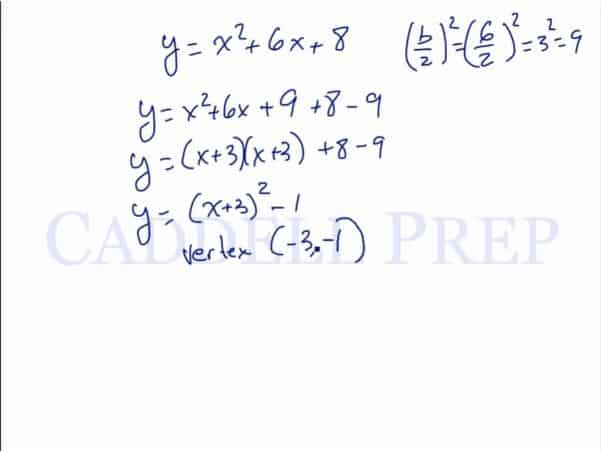In this video, we are going to look at the vertex form of a quadratic equation. After you finish this lesson, view all of our Algebra 1 lessons and practice problems.

If we look at a regular quadratic function such as$y=x^2$ and want to shift it to the right 3 units and down 4 units, then we know that the modified equation would be$y=(x-3)^2-4$

*Horizontal shifts are located within the parenthesis and vertical shifts are located outside of the parenthesis*

From this equation, we can clearly see that the vertex point is at (3,-4).

This form is very useful in identifying the coordinates of the vertex.

If we were given an equation in standard form, we can complete the square to get it to vertex form.
For example:$y=x^2+6x+8$
To solve by completing the square, we want to solve for the number to add by using$(\frac{b}{2})^2$
So:$(\frac{6}{2})^2=3^2=9$
This means that we have to add 9 to complete the square. However, if we add 9 then it changes the formula. To counteract that, we will also subtract 9.

Let’s focus on the first three terms in the equation.$y=x^2+6x+9+8-9$
When we factor those terms, we get$y=(x+3)(x+3)+8-9$
or$y=(x+3)^2-1$
Now, we have written the equation in vertex form. From here, we can see that the vertex is at (-3,-1).Examples of Vertex Form Of A Quadratic Equation

Example 1$y=x^2+10x+31$

To solve by completing the square, we want to solve for the number to add by using$(\frac{b}{2})^2$$(\frac{10}{2})^2=5^2=25$
This means that we have to add$25$ to complete the square. To counteract that, we will also subtract$25$.

Let’s focus on the first three terms in the equation.$y=x^2+10x+ 25+31-25$
When we factor those terms, we get$y=(x+5)(x+5)+31-25$
or$y=(x+5)^2+9$
Now, we have written the equation in vertex form. From here, we can see that the vertex is at$(-5,9)$.

Example 2$y=x^2+14x+36$

To solve by completing the square, we want to solve for the number to add by using$(\frac{b}{2})^2$$(\frac{14}{2})^2=7^2=49$
This means that we have to add$49$ to complete the square. To counteract that, we will also subtract$49$.

Let’s focus on the first three terms in the equation.$y=x^2+14x+49+36-49$
When we factor those terms, we get$y=(x+7)(x+7)+36-49$
or$y=(x+7)^2-7$
Now, we have written the equation in vertex form. From here, we can see that the vertex is at$(-7,-7)$.

Video-Lesson Transcript

Let’s go over vertex form of a quadratic equation using completing the square.

A basic quadratic function looks like a letter “U” with a form$y = x^2$

We could write this anywhere on the graph. Up, down, left, or right.

Now, if we want our line to be down by$4$ and move right by$3$, then we should also adjust the basic quadratic equation by the same number.

So now our equation is$y = (x - 3)^2 - 4$

From here, we can tell that the vertex is$(3, -4)$

But if we want to go left by$5$ and up by$2$, our new equation is$y = (x + 5)^2 + 2$

From here, you can tell that vertex is at$(-5, 2)$

Let’s do another one.

Let’s go up by$3$ and move right by$1$.

Here, our new equation is$y = (x - 1)^2 + 3$

And our vertex is$(1, 3)$

The equation form above is very useful in finding the vertex.

But what happens when you are given a standard equation like this:$y = x^2 + 5x + 4$

How do we translate this into the previous form?

We can do that by completing the square.

For example:$y = x^2 + 6x + 8$

Let’s transform this into the vertex form.

We want to complete the squares.

To do so, let’s use this formula for the$b$-term$\bigg( \dfrac{b}{2} \bigg)^2$$\bigg( \dfrac{6}{2} \bigg)^2$$(3)^2$$9$

Let’s continue completing the square$y = x^2 + 6x + 9 + 8 - 9$

Let’s just focus on the first three terms since it’s a perfect square.$y = (x + 3) (x + 3) + 8 - 9$$y = (x + 3)^2 - 1$

So now we arrive at the vertex form.

We can already identify the vertex$(-3, -1)$

Let’s look at one more$y = x^2 - 8x - 7$

Let’s solve the$b$-term first$\bigg( \dfrac{b}{2} \bigg)^2$$\bigg( \dfrac{8}{2} \bigg)^2$$(4)^2$$16$

Let’s go back to the equation and factor$y = x^2 - 8x + 16 - 7 - 16$$y = (x - 4)^2 - 23$

From this vertex form of a quadratic equation, we can already say that vertex is$(4, -23)$